# In-Place Algorithm

In-place has more than one definitions. One strict definition is.

An in-place algorithm is an algorithm that does not need an extra space and produces an output in the same memory that contains the data by transforming the input ‘in-place’. However, a small constant extra space used for variables is allowed.

In-place means that the algorithm does not use extra space for manipulating the input but may require a small though nonconstant extra space for its operation. Usually, this space is O(log n), though sometimes anything in o(n) (Smaller than linear) is allowed [Source : Wikipedia]

A Not In-Place Implementation of reversing an array

## C++

 `// An Not in-place C++ program to reverse an array ` `#include ` `using` `namespace` `std; ` `  `  `/* Function to reverse arr[] from start to end*/` `void` `revereseArray(``int` `arr[], ``int` `n) ` `{ ` `   ``// Create a copy array and store reversed ` `   ``// elements ` `   ``int` `rev[n]; ` `   ``for` `(``int` `i=0; i

## Java

 `// An Not in-place Java program ` `// to reverse an array ` `import` `java.util.*; ` ` `  `class` `GFG ` `{ ` `    ``/* Function to reverse arr[] ` `       ``from start to end*/` `    ``public` `static` `void` `revereseArray(``int` `[]arr,  ` `                                     ``int` `n) ` `    ``{ ` `        ``// Create a copy array ` `        ``// and store reversed ` `        ``// elements ` `        ``int` `[]rev = ``new` `int``[n]; ` `        ``for` `(``int` `i = ``0``; i < n; i++) ` `            ``rev[n - i - ``1``] = arr[i]; ` `         `  `        ``// Now copy reversed  ` `        ``// elements back to arr[] ` `        ``for` `(``int` `i = ``0``; i < n; i++) ` `            ``arr[i] = rev[i]; ` `    ``}  ` `     `  `    ``/* Utility function to ` `       ``print an array */` `    ``public` `static` `void` `printArray(``int` `[]arr,  ` `                                  ``int` `size) ` `    ``{ ` `    ``for` `(``int` `i = ``0``; i < size; i++) ` `        ``System.out.print(arr[i] + ``" "``); ` `    ``System.out.println(``""``); ` `    ``}  ` `     `  `    ``// Driver code ` `    ``public` `static` `void` `main(String[] args)  ` `    ``{ ` `        ``int` `arr[] = {``1``, ``2``, ``3``, ``4``, ``5``, ``6``};  ` `        ``int` `n = arr.length; ` `        ``printArray(arr, n);  ` `        ``revereseArray(arr, n);  ` `        ``System.out.println(``"Reversed array is"``); ` `        ``printArray(arr, n);  ` `    ``} ` `} ` ` `  `// This code is contributed ` `// by Harshit Saini `

## Python3

 `# An Not in-place Python program  ` `# to reverse an array ` ` `  `''' Function to reverse arr[] ` `    ``from start to end '''` `def` `revereseArray(arr, n): ` `     `  `    ``# Create a copy array  ` `    ``# and store reversed ` `    ``# elements ` `    ``rev ``=` `n ``*` `[``0``] ` `    ``for` `i ``in` `range``(``0``, n): ` `        ``rev[n ``-` `i ``-` `1``] ``=` `arr[i] ` `             `  `    ``# Now copy reversed ` `    ``# elements back to arr[] ` `    ``for` `i ``in` `range``(``0``, n): ` `        ``arr[i] ``=` `rev[i] ` `         `  `# Driver code ` `if` `__name__ ``=``=` `"__main__"``: ` `    ``arr ``=` `[``1``, ``2``, ``3``, ``4``, ``5``, ``6``] ` `    ``n ``=` `len``(arr) ` `    ``print``(``*``arr)  ` `    ``revereseArray(arr, n);  ` `    ``print``(``"Reversed array is"``) ` `    ``print``(``*``arr)  ` `     `  `# This code is contributed ` `# by Harshit Saini `

## C#

 `// An Not in-place C# program ` `// to reverse an array ` `using` `System; ` ` `  `class` `GFG ` `{ ` `    ``/* Function to reverse arr[] ` `    ``from start to end*/` `    ``public` `static` `void` `revereseArray(``int``[] arr,  ` `                                    ``int` `n) ` `    ``{ ` `        ``// Create a copy array ` `        ``// and store reversed ` `        ``// elements ` `        ``int``[] rev = ``new` `int``[n]; ` `        ``for` `(``int` `i = 0; i < n; i++) ` `            ``rev[n - i - 1] = arr[i]; ` `         `  `        ``// Now copy reversed  ` `        ``// elements back to arr[] ` `        ``for` `(``int` `i = 0; i < n; i++) ` `            ``arr[i] = rev[i]; ` `    ``}  ` `     `  `    ``/* Utility function to ` `    ``print an array */` `    ``public` `static` `void` `printArray(``int``[] arr,  ` `                                ``int` `size) ` `    ``{ ` `    ``for` `(``int` `i = 0; i < size; i++) ` `        ``Console.Write(arr[i] + ``" "``); ` `    ``Console.Write(``"\n"``); ` `    ``}  ` `     `  `    ``// Driver code ` `    ``public` `static` `void` `Main()  ` `    ``{ ` `        ``int``[] arr = {1, 2, 3, 4, 5, 6};  ` `        ``int` `n = arr.Length; ` `        ``printArray(arr, n);  ` `        ``revereseArray(arr, n);  ` `        ``Console.WriteLine(``"Reversed array is"``); ` `        ``printArray(arr, n);  ` `    ``} ` `} ` ` `  `// This code is contributed by Ita_c. `

Output:

```1 2 3 4 5 6
Reversed array is
6 5 4 3 2 1
```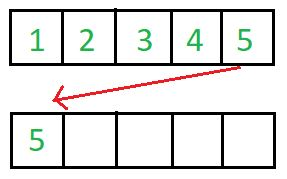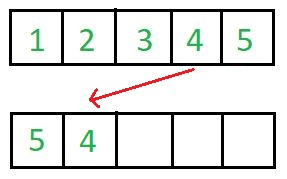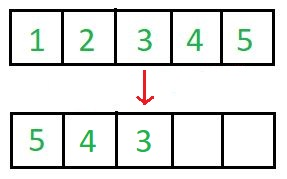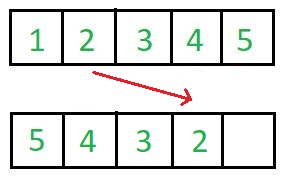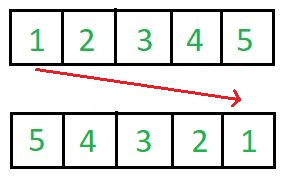This needs O(n) extra space and is an example of not-in-place algorithm.

An In-Place Implementation of Reversing an array.

## C++

 `// An in-place C++ program to reverse an array ` `#include ` `using` `namespace` `std; ` `  `  `/* Function to reverse arr[] from start to end*/` `void` `revereseArray(``int` `arr[], ``int` `n) ` `{ ` `   ``for` `(``int` `i=0; i

## Java

 `// An in-place Java program ` `// to reverse an array ` `import` `java.util.*; ` ` `  `class` `GFG ` `{ ` `    ``public` `static` `int` `__(``int` `x, ``int` `y) {``return` `x;} ` `     `  `    ``/* Function to reverse arr[]  ` `       ``from start to end*/` `    ``public` `static` `void` `revereseArray(``int` `[]arr,  ` `                                     ``int` `n) ` `    ``{ ` `        ``for` `(``int` `i = ``0``; i < n / ``2``; i++) ` `            ``arr[i] = __(arr[n - i - ``1``],  ` `                        ``arr[n - i - ``1``] = arr[i]); ` `    ``}  ` `     `  `    ``/* Utility function to  ` `       ``print an array */` `    ``public` `static` `void` `printArray(``int` `[]arr,  ` `                                  ``int` `size) ` `    ``{ ` `        ``for` `(``int` `i = ``0``; i < size; i++) ` `            ``System.out.print(Integer.toString(arr[i]) + ``" "``);  ` `        ``System.out.println(``""``); ` `    ``}  ` `     `  `    ``// Driver code ` `    ``public` `static` `void` `main(String[] args)  ` `    ``{ ` `        ``int` `[]arr = ``new` `int``[]{``1``, ``2``, ``3``, ``4``, ``5``, ``6``};  ` `        ``int` `n = arr.length; ` `        ``printArray(arr, n);  ` `        ``revereseArray(arr, n);  ` `        ``System.out.println(``"Reversed array is"``); ` `        ``printArray(arr, n);  ` `    ``} ` `} ` ` `  `// This code is contributed  ` `// by Harshit Saini `

## Python3

 `# An in-place Python program ` `# to reverse an array ` ` `  `''' Function to reverse arr[] ` `    ``from start to end'''` `def` `revereseArray(arr, n): ` `     `  `    ``for` `i ``in` `range``(``0``, ``int``(n ``/` `2``)): ` `        ``arr[i], arr[n ``-` `i ``-` `1``] ``=` `arr[n ``-` `i ``-` `1``], arr[i] ` ` `  ` `  `# Driver code ` `if` `__name__ ``=``=` `"__main__"``: ` `     `  `    ``arr ``=` `[``1``, ``2``, ``3``, ``4``, ``5``, ``6``] ` `    ``n ``=` `len``(arr) ` `    ``print``(``*``arr) ` `    ``revereseArray(arr, n)  ` `    ``print``(``"Reversed array is"``) ` `    ``print``(``*``arr) ` `     `  `# This code is contributed  ` `# by Harshit Saini `

## C#

 `// An in-place C# program ` `// to reverse an array ` `using` `System;  ` `     `  `class` `GFG ` `{ ` `    ``public` `static` `int` `__(``int` `x, ``int` `y) {``return` `x;} ` `     `  `    ``/* Function to reverse arr[]  ` `    ``from start to end*/` `    ``public` `static` `void` `revereseArray(``int` `[]arr,  ` `                                    ``int` `n) ` `    ``{ ` `        ``for` `(``int` `i = 0; i < n / 2; i++) ` `            ``arr[i] = __(arr[n - i - 1],  ` `                        ``arr[n - i - 1] = arr[i]); ` `    ``}  ` `     `  `    ``/* Utility function to  ` `    ``print an array */` `    ``public` `static` `void` `printArray(``int` `[]arr,  ` `                                ``int` `size) ` `    ``{ ` `        ``for` `(``int` `i = 0; i < size; i++) ` `            ``Console.Write(arr[i] + ``" "``);  ` `        ``Console.WriteLine(``""``); ` `    ``}  ` `     `  `    ``// Driver code ` `    ``public` `static` `void` `Main(String[] args)  ` `    ``{ ` `        ``int` `[]arr = ``new` `int``[]{1, 2, 3, 4, 5, 6};  ` `        ``int` `n = arr.Length; ` `        ``printArray(arr, n);  ` `        ``revereseArray(arr, n);  ` `        ``Console.WriteLine(``"Reversed array is"``); ` `        ``printArray(arr, n);  ` `    ``} ` `} ` ` `  `/* This code is contributed by PrinciRaj1992 */`

Output:

```1 2 3 4 5 6
Reversed array is
6 5 4 3 2 1
```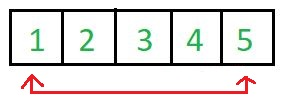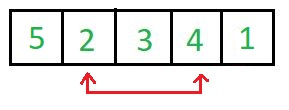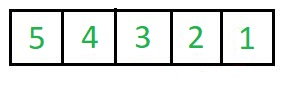This needs O(1) extra space for exchanging elements and is an example of in-place algorithm.

Which Sorting Algorithms are In-Place and which are not?
In Place : Bubble sort, Selection Sort, Insertion Sort, Heapsort.

Not In-Place : Merge Sort. Note that merge sort requires O(n) extra space.

What about QuickSort? Why is it called In-Place?
QuickSort uses extra space for recursive function calls. It is called in-place according to broad definition as extra space required is not used to manipulate input, but only for recursive calls.

My Personal Notes arrow_drop_upCheck out this Author's contributed articles.

If you like GeeksforGeeks and would like to contribute, you can also write an article using contribute.geeksforgeeks.org or mail your article to contribute@geeksforgeeks.org. See your article appearing on the GeeksforGeeks main page and help other Geeks.

Please Improve this article if you find anything incorrect by clicking on the "Improve Article" button below.